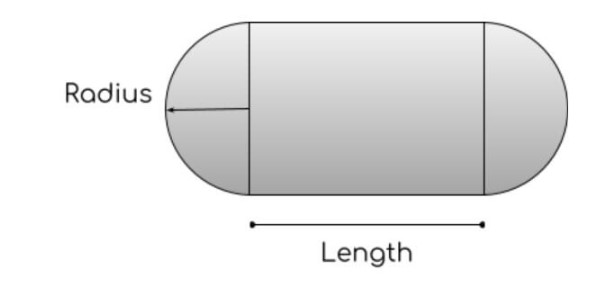# Cylinder with hemispherical ends Calculator (Volume)

To find volume of hemisphere, write radius & length into input boxes and click calculate button

Formula:
V = πr2ℓ + 43 πr3
cm
cm

Give Us Feedback

## Volume of Hemisphere Calculator

The cylinder with hemispherical ends calculator is a physics tool that helps to find the volume of a particular type of cylinder. It needs only two values to calculate the volume.

## How to use this calculator?

The instructions to use this tool are:

1. Enter the radius of the hemispheres.
2. Enter the length of the cylinder.
3. Click Calculate.

That’s all you need to do. The calculator will give the calculated answer within a fraction of a second. Also, try our Cylinder tank Calculator

## Cylinder with hemispherical ends:

It is actually a shape in which two halves of a sphere aka hemispheres are linked by an equator or cylinder. It is also known as a capsule.It has a vast use in biomedical sciences.

## Formula for the volume of a capsule/cylinder with hemispherical ends:

The volume of a capsule is calculated by using the formula:

= (π * r2 * h) + (( 4 / 3 ) * π * r3)

Where h is length and r is radius.

Example:

A sphere has a radius of 3.5 cm. What will be the volume of the capsule made using this sphere? Consider the length as 30cm.

Solution:

Step 1: Write the given data.

Length = 30cm

Step 2: Put the values in the formula.

= (π * r2 * h) + (( 4 / 3 ) * π * r3)

= (π * (3.5)2 * 30) + (( 4 / 3 ) * π* (3.5)3)

Step 3: Solve.

= (π * (3.5)2 *30) + (( 4 / 3 ) * π * (3.5)3

= (π * (3.5)2 *30) + (( 4 / 3 ) * π * (3.5)3

= (1154.5) + (179.6)

= 1334.1

### Math Tools